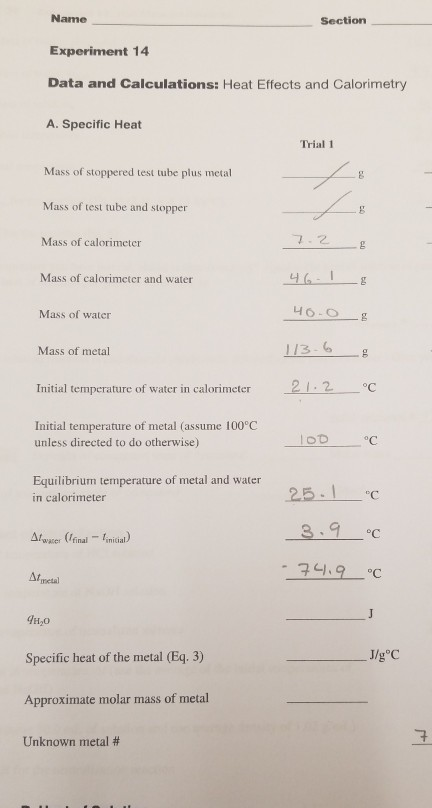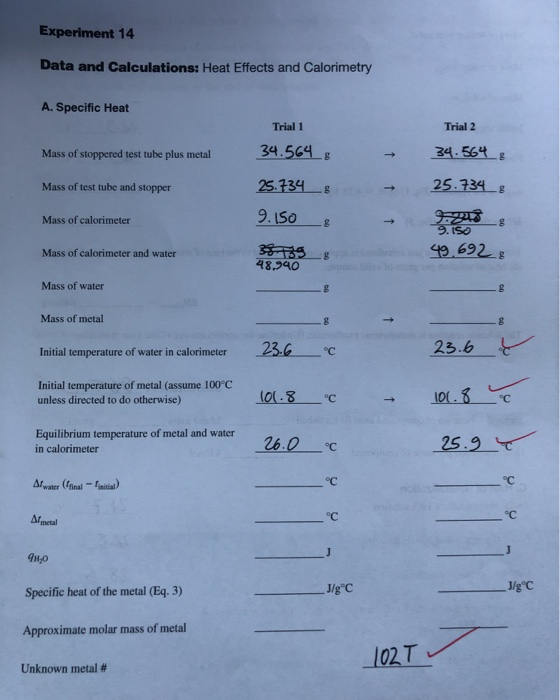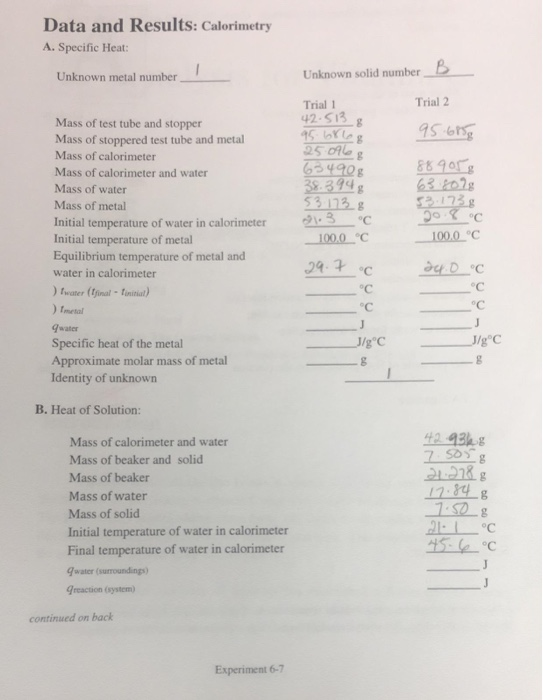# PART B Watch the Specific Heat video (7 minutes) below. https://www.youtube.com/watch?v=HPHee WK4kik Data for the experiment...PART B Watch the Specific Heat video (7 minutes) below. https://www.youtube.com/watch?v=HPHee WK4kik Data for the experiment has been provided below. Two Trials were done with the same metal sample. One using room temperature water in the calorimeter and one using cold water in the calorimeter. Perform the calculations needed complete the table and identify the metal sample. Be sure to report your answers using the correct number of significant figures.
Data & Calculations Trial 2 Cold Water in Calorimeter Trial 1 Room Temp. Water in Calorimeter 24.30 g 1. Mass of metal sample 24.30 g 6.54 g 6.54 g 2. Mass of calorimeter (Styrofoam cup) 3. Mass of calorimeter + water 38.50 g 66.92 g 4. Mass of water 5. Initial water temperature 22.8 °C 15.2 °C 99.9 °C 99.1 °C 6. Temperature of heated metal sample 25.5 °C 17.1 °C 7. Final temperature of water and metal 8. Change in temperature (ATw) of the water in the calorimeter 9. Change in temperature (ATM) of the metal sample 10. Specific heat (sp bt) of water 4.184 J/gºS 4.184 J/g °C 11. Heat (gw) gained by water 12. Heat (qm) lost by metal 13. Specific heat (sp ht) of the metal (calculated value) 14. Average of Trial 1 & 2 Specific Heat Results 15. Metal identity 16. Theoretical Specific Heat (from Table 1) 17. % error (Recall formula from Lab 1)##### Add Answer of: PART B Watch the Specific Heat video (7 minutes) below. https://www.youtube.com/watch?v=HPHee WK4kik Data for the experiment...
Similar Homework Help Questions
• ### Name Section Experiment 14 Data and Calculations: Heat Effects and Calorimetry A. Specific Heat Trial 1...Name Section Experiment 14 Data and Calculations: Heat Effects and Calorimetry A. Specific Heat Trial 1 Mass of stoppered test tube plus metal Mass of test tube and stopper Mass of calorimeter 7.2 g Mass of calorimeter and water 46-1 Mass of water 40-0 Mass of metal 113.6 21.2 . °c Initial temperature of water in calorimeter Initial temperature of metal (assume 100°C unless directed to do otherwise) 100 °c Equilibrium temperature of metal and water in calorimeter 25.1 3.9...

• ### Experiment: Specific heat capacity Aim: To determine the specific heat capacity of a solid Using the...

Experiment: Specific heat capacity Aim: To determine the specific heat capacity of a solid Using the attached data, calculate the following: Mass of water Specific heat capacity of copper (experimental value) % Error (using the theoretical value of the specific heat capacity of copper, 390 J/kg/K) METHOD: Fill the steam generator more than halfway with water, bring the water to boil. Weigh the mass of all three different metal solid, and record them on the table of results. Weigh the...

• ### find missing blanks please! Experiment 14 Data and Calculations: Heat Effects and Calorimetry A.Specific Heat Trial...find missing blanks please! Experiment 14 Data and Calculations: Heat Effects and Calorimetry A.Specific Heat Trial 1 Trial 2 34.564& 34.56 g Mass of stoppered test tube plus metal 25.734 g 25.734 Mass of test tube and stopper 9.1S0 Mass of calorimeter 9.ISo 4.692 8 Mass of calorimeter and water 48,940 Mass of water Mass of metal 23.6 23.6 C Initial temperature of water in calorimeter Initial temperature of metal (assume 100°C unless directed to do otherwise) Equilibrium temperature of...

• ### Specific Heat Capacity

A coffee cup calorimeter, Ccal=1.639×103 J°C-1, contains 30.6 mL of wtaer at a temperature of 18.5°C. An unknown metal with amass of 48.30 g is heated to 69.6°C then added to the calorimeter. The final temperature of the calorimeter is 29.4°C. What is the specific heat capacity of theunknown metal?The specific heat capacity of water is 4.184 J g-1°C.

• ### heat lost, heat gained, specific heat

Mass of calorimeter and stirrer m_1_ = 76 gMass of water + calorimeter + stirrer m_2_ = 268 gMass of water m_w_ = m_1_ – m_2_ = 192 gInitial temperature of water and calorimeter T_wi_ = 23.3 degree CelsiusMass of metal sample m_x_ = 56.1 gInitial temperatures of metal sample T_xi_ = 97 degree CelsiusFinal temperature of water, calorimeter, and sample T_f_ = 25.3 degree Celsiuswhat is the heat lost, heat gained, and specific heat of the metal sample?

• ### In this experiment a hot metal block at 100oC is added to cold water in a calorimeter

In this experiment a hot metal block at 100oC is added to cold water in a calorimeter. The heat lost by the metal block equals the heat gained by the water and the two end up at the same temperature. In one experiment, the mass of the metal block is 89.5 grams, the volume of water used is 75.6 mL, the temperature of the cold water extrapolated to the time of mixing is 18.2oC, and the temperature of the metal...

• ### 1. An unknown sample is being evaluated in lab. What is the specific heat of the...

1. An unknown sample is being evaluated in lab. What is the specific heat of the compound if it requires 105.06 J to raise the temperature of 51.68 grams of the unknown from 15.25 °C to 22.9 °C. 2. A 25.00 gram sample of an unknown metal initially at 99.0 degrees Celsius is added to 50.00 grams of water initially at 5.94 degrees Celsius. The final temperature of the system is 20.15 degrees Celsius. Calculate the specific heat of the...

• ### 1. An unknown sample is being evaluated in lab. What is the specific heat of the...

1. An unknown sample is being evaluated in lab. What is the specific heat of the compound if it requires 105.06 J to raise the temperature of 51.68 grams of the unknown from 15.25 °C to 22.9 °C. 2. A 25.00 gram sample of an unknown metal initially at 99.0 degrees Celsius is added to 50.00 grams of water initially at 5.94 degrees Celsius. The final temperature of the system is 20.15 degrees Celsius. Calculate the specific heat of the...

• ### Data and Results: Calorimetry A. Specific Heat: Unknown metal number Unknown solid number Trial 2...Data and Results: Calorimetry A. Specific Heat: Unknown metal number Unknown solid number Trial 2 Trial 1 42.SI3 Mass of test tube and stopper Mass of stoppered test tube and metal Mass of calorimeter Mass of calorimeter and water Mass of water Mass of metal Initial temperature of water in calorimeter Initial temperature of metal Equilibrium temperature of metal and water in calorimeter 38.394g 53 13 g 63 to2g 0o C water (inal-onitiat oC oC Imetal qwater Specific heat of...

• ### specific heat

A 250.0g sample of metal shot is heated to a temperature of 98 degrees Celcius. It is placed in 100.0g of water in a brass calorimeter cup with a brass stirrer.The total mass of the cup and the stirrer is 50.0g. The initial temperature of the water, stirrer, and calorimeter cup is 20.0 Celcius. The final equilibriumtemperature of the system is 30.0 degrees Celcius. What is the specific heat of the metal sample? ( The specific heat of brass is...

Free Homework App